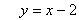Year 8 Interactive Maths - Second Edition

## Linear Patterns

If the plotted points make a pattern, then the coordinates of each point may have the same relationship between the x and y values.  In such a case, the x and y values are connected by a certain rule.

A linear pattern is said to exist when the points examined form a straight line.

#### Example 2

a.  Plot the points in the following table on a Cartesian plane.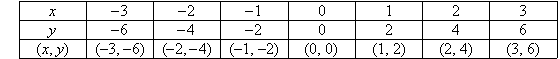b.  Describe the pattern in words.
c.  State the next point in the pattern.
d.  Find the rule that connects x and y.

##### Solution: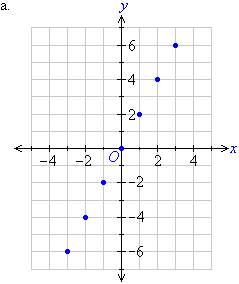b.  A linear pattern exists as the points form a straight line.  The pattern shows that as the x value increases by 1, the y value increases by 2.

c.  The next x value will be 4 and the next y value will be 8.  So, the next point is (4, 8).

d.  By trial and error, we look for a relationship between the values of x and y.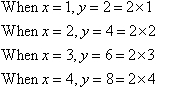The above information for x and y indicates that the y-coordinate is twice the x-coordinate.   This means y is twice x.  So, the rule that connects the x-coordinate and the y-coordinate is: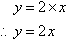#### Example 3

a.  Plot the following points on the Cartesian plane:
(–3, –9), (–2, –6), (–1, –3),  (0, 0), (1, 3), (2, 6), (3, 9)

b.  Describe the pattern in words.
c.  Find the rule that connects x and y.

##### Solution: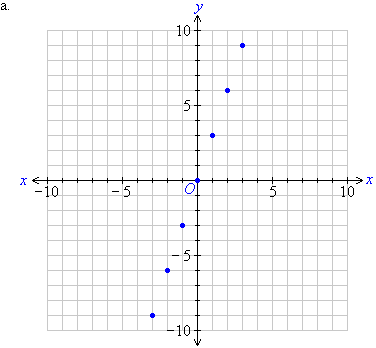b.  We notice that the points plotted on the Cartesian plane form a straight line.  So, a linear pattern exists.  We can describe this pattern in words as follows:

The  y-coordinate is three times the x-coordinate.  This means y is three times x.

c.  The rule that connects the x-coordinate and y-coordinate is: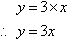#### Example 4

a.  Plot the following points on the Cartesian plane:
(–5, –7), (–4, –6), (–3, –5), (–2, –4), (–1, –3), (0, –2), (1, –1), (2, 0), (3, 1), (4, 2), (5, 3), (6, 4)

b.  Describe the pattern in words.
c.  Find the rule that connects x and y.

##### Solution: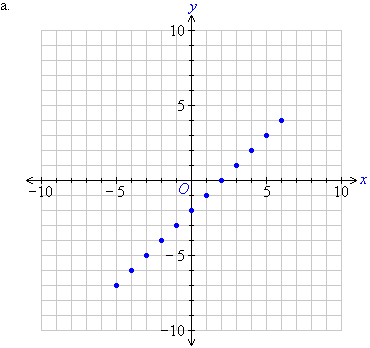b.  We notice that the points plotted on the Cartesian plane form a straight line.  So, a linear pattern exists.  We can describe this pattern in words as follows:

The y-coordinate is two less than the x-coordinate.

c.  The rule that connects the x-coordinate and the y-coordinate is: